# Irodov Solutions: Scattering of Particles: Rutherford-Bohr Atom - 2 Notes | Study I. E. Irodov Solutions for Physics Class 11 & Class 12 - JEE

## JEE: Irodov Solutions: Scattering of Particles: Rutherford-Bohr Atom - 2 Notes | Study I. E. Irodov Solutions for Physics Class 11 & Class 12 - JEE

The document Irodov Solutions: Scattering of Particles: Rutherford-Bohr Atom - 2 Notes | Study I. E. Irodov Solutions for Physics Class 11 & Class 12 - JEE is a part of the JEE Course I. E. Irodov Solutions for Physics Class 11 & Class 12.
All you need of JEE at this link: JEE

Q.17. The effective cross section of a gold nucleus corresponding to the scattering of monoenergetic alpha particles within the angular interval from 90° to 180° is equal to Δσ = 0.50 kb. Find:
(a) the energy of alpha particles;
(b) the differential cross section of scattering dσ/dΩ (kb/sr) corresponding to the angle θ = 60°.

Ans. (a) From the previous formula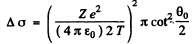or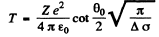Substituting the values with Z = 79 we get (θO = 90°)

T = 0.903 MeV

(b) The differential scattering cross section is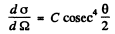where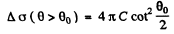Thus from the given data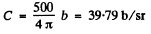So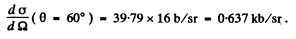Q.18. In accordance with classical electrodynamics an electron moving with acceleration ω loses its energy due to radiation as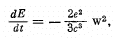where e is the electron charge, c is the velocity of light. Estimate the time during which the energy of an electron performing almost harmonic oscillations with frequency ω = 5.1015  s-1  will decrease η = 10 times.

Ans. The formula in MKS units is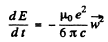For an electron performing (linear) harmonic vibrations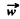is in some definite directions with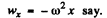Thus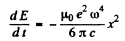If the radiation loss is small (i.e. if ω is not too large), then the motion of the electron is always close to simple harmonic with slowly decreasing amplitude. Then we can write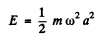and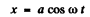and average the above equation ignoring the variation of a in any cycle. Thus we get the equation, on using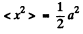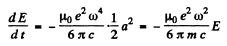since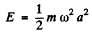for a harmonic oscillator.

This equation integrates to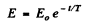where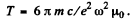It is then seen that energy decreases η times in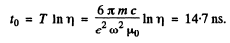Q.19. Making use of the formula of the foregoing problem, estimate the time during which an electron moving in a hydrogen atom along a circular orbit of radius r = 50 pm would have fallen onto the nucleus. For the sake of simplicity assume the vector ω to be permanently directed toward the centre of the atom.

Ans. Moving around the nucleus, the electron radiates and its energy decreases. This means that the electron gets nearer the nucleus. By the statement of the problem we can assume that the electron is always moving in a circular orbit and the radial acceleration by Newton’s law is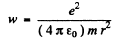directed inwards. Thus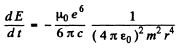On the other hand in a circular orbit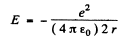so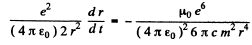or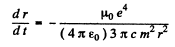Integrating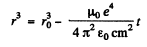and the radius fells to zero in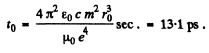Q.20. Demonstrate that the frequency ω of a photon emerging when an electron jumps between neighbouring circular orbits of a hydrogen-like ion satisfies the inequality ωn > ω > ωn +1, where ωn  and ωn +1 are the frequencies of revolution of that electron around the nucleus along the circular orbits. Make sure that as n   →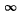the frequency of the photon ω → ωn.

Ans. In a circular orbit we have the following formula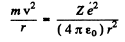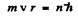Then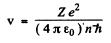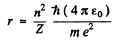The energy E is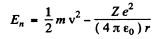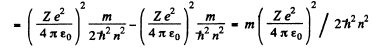and the circular frequency of this orbit is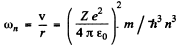On the other hand the frequency co of the light emitted when the electron makes a transition n + 1 →  n is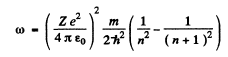Thus the inequality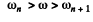will result if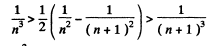Or multiplying by n2(n + 1)2 we have to prove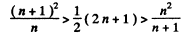This can be written as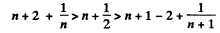This is obvious because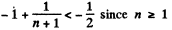For laige n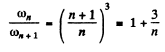so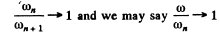Q.21. A particle of mass m moves along a circular orbit in a centrosymmetrical potential field U (r) = kr212. Using the Bohr quantization condition, find the permissible orbital radii and energy levels of that particle.

Ans. We have the following equation (we ignore reduced mass effects)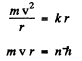so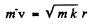and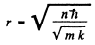and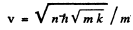The energy levels are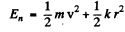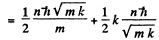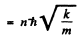Q.22. Calculate for a hydrogen atom and a He + ion:
(a) the radius of the first Bohr orbit and the velocity of an electron moving along it;
(b) the kinetic energy and the binding energy of an electron in the ground state;
(c) the ionization potential, the first excitation potential and the wavelength of the resonance line (n' = 2  →  n = 1).

Ans. The basic equations have been derived in the problem (6.20). We rewrite them here and determine the the required values.

(a)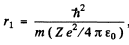Thus Z = 1 for H , Z = 2 for He+

Thus                     r1 = 52.8 pm, for H atom
r= 26-.4 pm, for He+ ion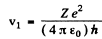v= 2.191 x 106 m/s for H atom

= 4.382 x 106 m/s for He+ ion

(b)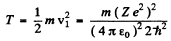T = 13.65 eV for H atom T = 54.6 eV for He+ ion

In both cases E h = T because Eb = - E and E = - T (Recall that for coulomb force V = - 2 T )

(c) The ionization potential φ is given by

= E

so φ = 13.65 volts for H atom

φ= 54.6 volts for He+ ion 13.65

The energy levels are

The energy levels are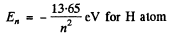for H atom

and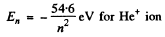Thus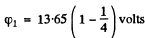= 10.23 volts for H atom

= 4 x 10.23 = 40.9 volts for He+ ion

The wavelength of the resonance line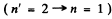is given by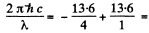10.23 eV for H atom

so   λ = 121.2 nm for H atom

For He+ ion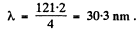Q.23. Calculate the angular frequency of an electron occupying the second Bohr orbit of He+ ion.

Ans. This has been calculated before in problem (6.20). It is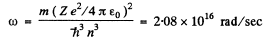Q.24. For hydrogen-like systems find the magnetic moment tt n corresponding to the motion of an electron along the n-th orbit and the ratio of the magnetic and mechanical moments µn /Mn. Calculate the magnetic moment of an electron occupying the first Bohr orbit.

Ans. An electron moving in a circle with a time period T constitutes a current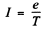and forms a current loop of area πr2. This is equivalent to magnetic moment,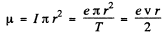on using v = 2πr/T. Thus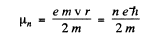for the nth orbit. (In Gaussian units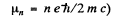We see that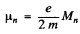where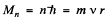is the angular momentum

Thus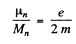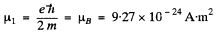(In CGS units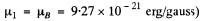Q.25. Calculate the magnetic field induction at the centre of a hydrogen atom caused by an electron moving along the first Bohr orbit.

Ans. The revolving electron is equivalent to a circular current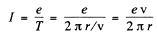The magnetic induction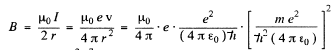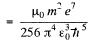Substitution gives B = 12.56 T at the centre. (In Gaussian units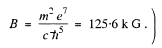Q.26. Calculate and draw on the wavelength scale the spectral intervals in which the Lyman, Balmer, and Paschen series for atomic hydrogen are confined. Show the visible portion of the spectrum.

Ans. From the general formula for the transition n2 → n1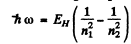where EH ≥ 13.65eV. Then

(1) Lyman, n= 1, n2 = 2,3. Thus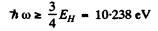This conesponds to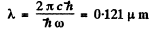and Lyman lines have λ ≤ 0.121μm with the series limit at .0909μm

(2) Balmer : n2 = 2 , n3 = 3, 4 ,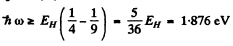This corresponds to
λ = 0.65 μm

and Balmer series has λ ≤ 0.65μm with the series limit at X = 0.363μm

(3) Paschen : n2 = 3 , n= 4, 5,...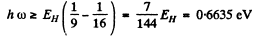This corresponds to λ = 1.869μm

with the series limit at λ = 0.818μm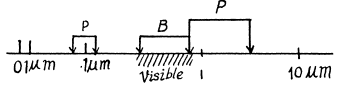Q.27. To what series does the spectral line of atomic hydrogen belong if its wave number is equal to the difference between the wave numbers of the following two lines of the Balmer series: 486.1 and 410.2 nm? What is the wavelength of that line?

Ans. The Balmer line of wavelength 486-1 nm is due to the transition 4 → 2 while the Balmer line of wavelingth 4→2 nm is due to the transition 6→2. The line whose wave number corresponds to the difference in wave numbers of these two lines is due to the transition 6 → 4. That line belongs to the Brackett series. The wavelength of this line is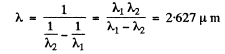Q.28. For the case of atomic hydrogen find:
(a) the wavelengths of the first three lines of the Balmer series;
(b) the minimum resolving power λ/δλ of a spectral instrument capable of resolving the first 20 lines of the Balmer series.

Ans. The energies are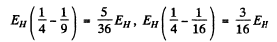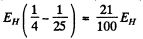They correspond to wavelengths 654.2 n m , 484.6 n m and 433 nm

The nth line of the Balmer series has the energy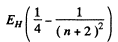For n = 19, we get the wavelength 366.7450 nm

For n = 20 we get the wavelength 366.4470 nm

To resolve these lines we require a resolving power of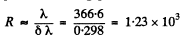Q.29. Radiation of atomic hydrogen falls normally on a diffraction grating of width l = 6.6 mm. The 50th line of the Balmer series in the observed spectrum is close to resolution at a diffraction angle θ (in accordance with Rayleigh's criterion). Find that angle.

Ans. For the Balmer series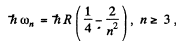where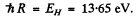Thus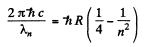or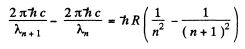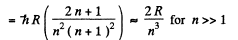Thus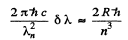or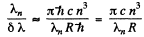On the other hand for just resolution in a diffraction grating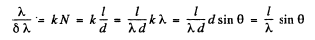Hence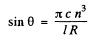Substitution gives θ = 59.4°.

Q.30. What element has a hydrogen-like spectrum whose lines have wavelengths four times shorter than those of atomic hydrogen?

Ans. If all wavelengths are four times shorter but otherwise similar to the hydrogen atom spectrum then the energy levels of the given atom must be four times greater.

This means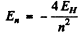compared to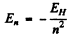for hydrogen atom. Therefore the spectrum is that o f He+ ion (Z = 2).

Q.31. How many spectral lines are emitted by atomic hydrogen excited to the n-th energy level?

Ans. Because of cascading all possible transitions are seen. Thus we look for the number of ways in which we can select upper and lower levels. The number of ways we can do this is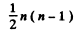where the factor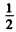takes account of the fact that the photon emission always arises from upper lower transition.

Q.32. What lines of atomic hydrogen absorption spectrum fall within the wavelength range from 94.5 to 130.0 nm?

Ans. These are the Lyman lines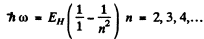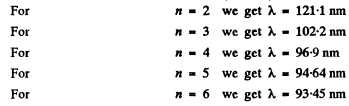Thus at the level of accuracy of our calculation, there are four lines 121.1nm , 102.2nm , 96.9nm and 94.64nm .

The document Irodov Solutions: Scattering of Particles: Rutherford-Bohr Atom - 2 Notes | Study I. E. Irodov Solutions for Physics Class 11 & Class 12 - JEE is a part of the JEE Course I. E. Irodov Solutions for Physics Class 11 & Class 12.
All you need of JEE at this link: JEEUse Code STAYHOME200 and get INR 200 additional OFF

## I. E. Irodov Solutions for Physics Class 11 & Class 12

111 docs

Track your progress, build streaks, highlight & save important lessons and more!

,

,

,

,

,

,

,

,

,

,

,

,

,

,

,

,

,

,

,

,

,

;